## How to solve indefinite integral of 3 x square plus 6 x minus 4 divided by x by dx ?

Short answer: indefinite integral of 3 x square plus 6 x minus 4 divided by x by dx is 98x+C. ∫ ((3x^2 +6x-4)/x)dx is not particulary hard integral. You can solve it in 8 easy steps. We will walk you through and explain everything. Let's start.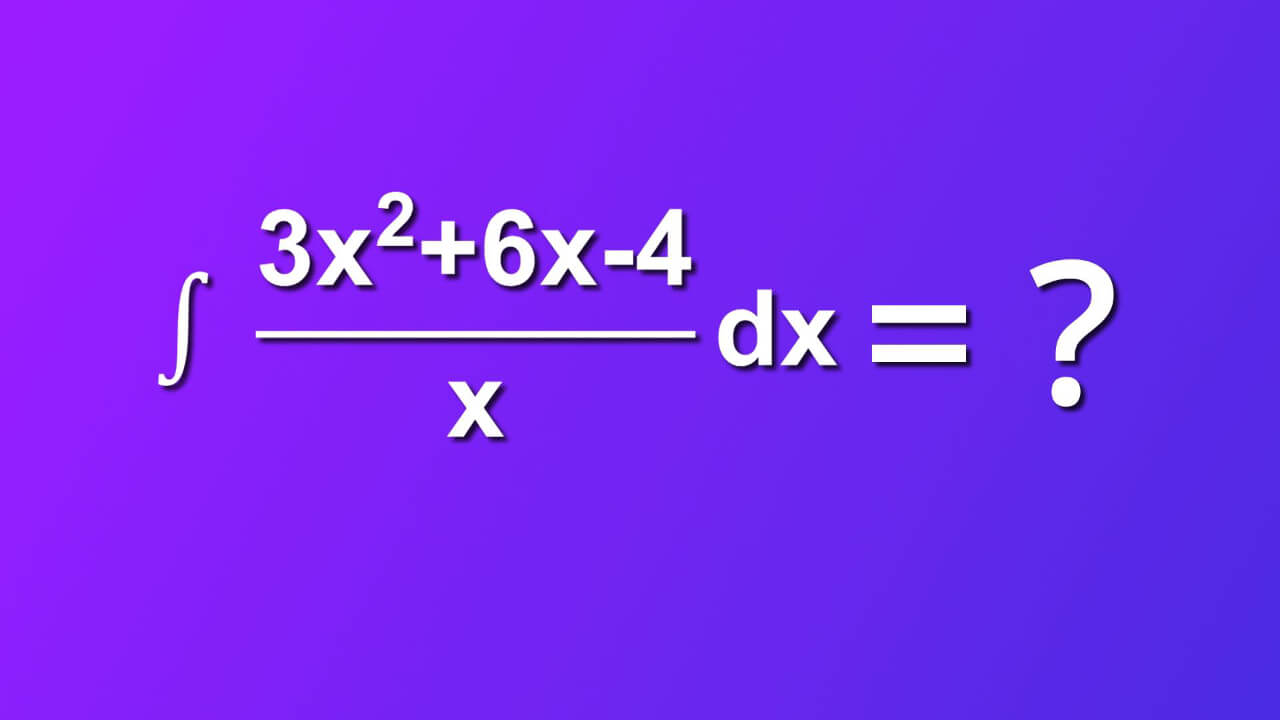## Required assumtions

Usually, we have some additional info about function f of (x). In our case:

1. f(x) belongs to real numbers
2. f(x) is integrable in that domain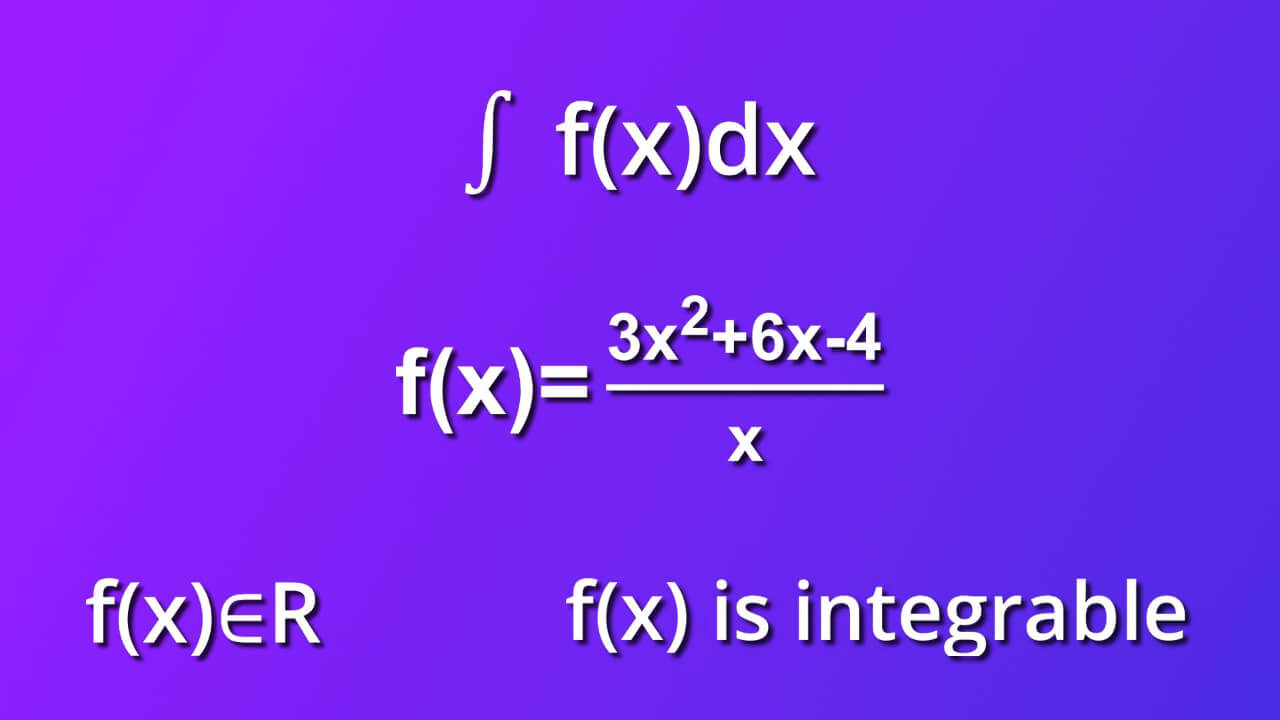## Step by step solution of ∫ ((3x^2 +6x-4)/x)dx

We will solve ∫ ((3x^2 +6x-4)/x)dx in 8 easy steps. Let's get started

### Step 1

From symbol dx we know that differential of variable x indicates that the variable of integration is x. First, let’s rewrite our equation to get three separate ones.### Step 2

We’ve got 3 x square divided by x plus 6x divided by x minus 4 divided by x.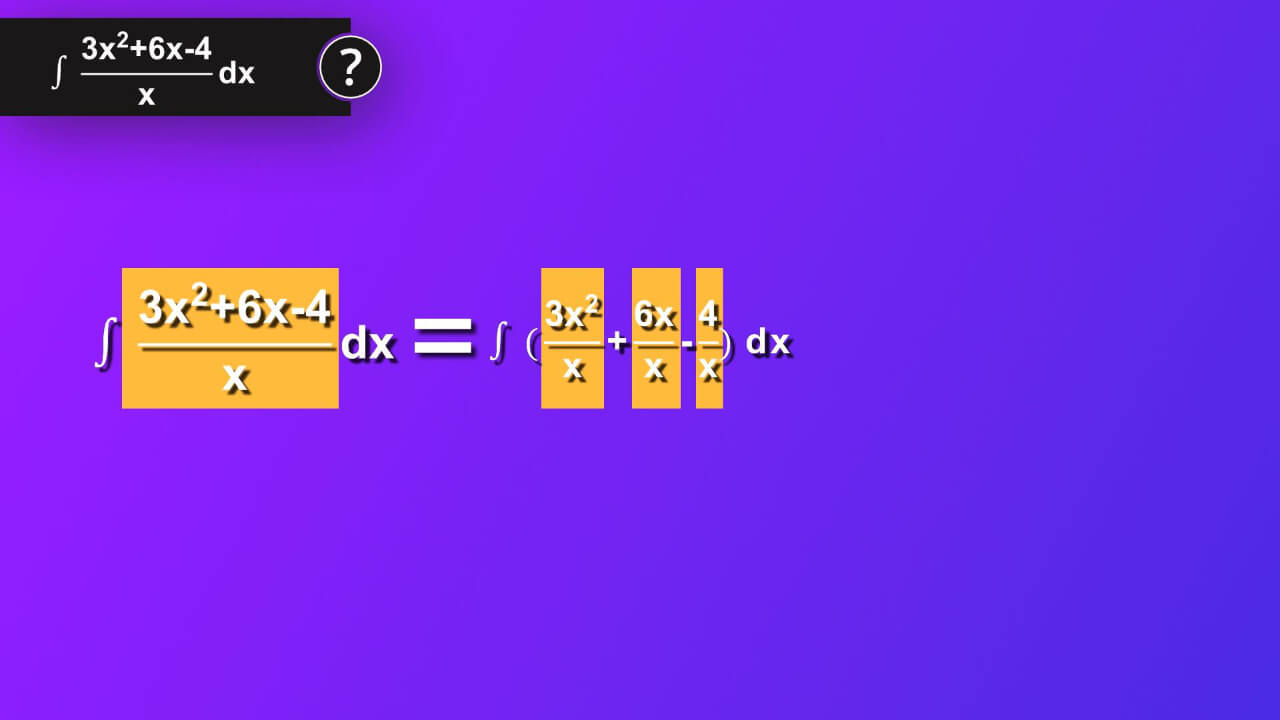### Step 3

So, we’ve got 3x plus 6 minus 4 divided by x as our integrand.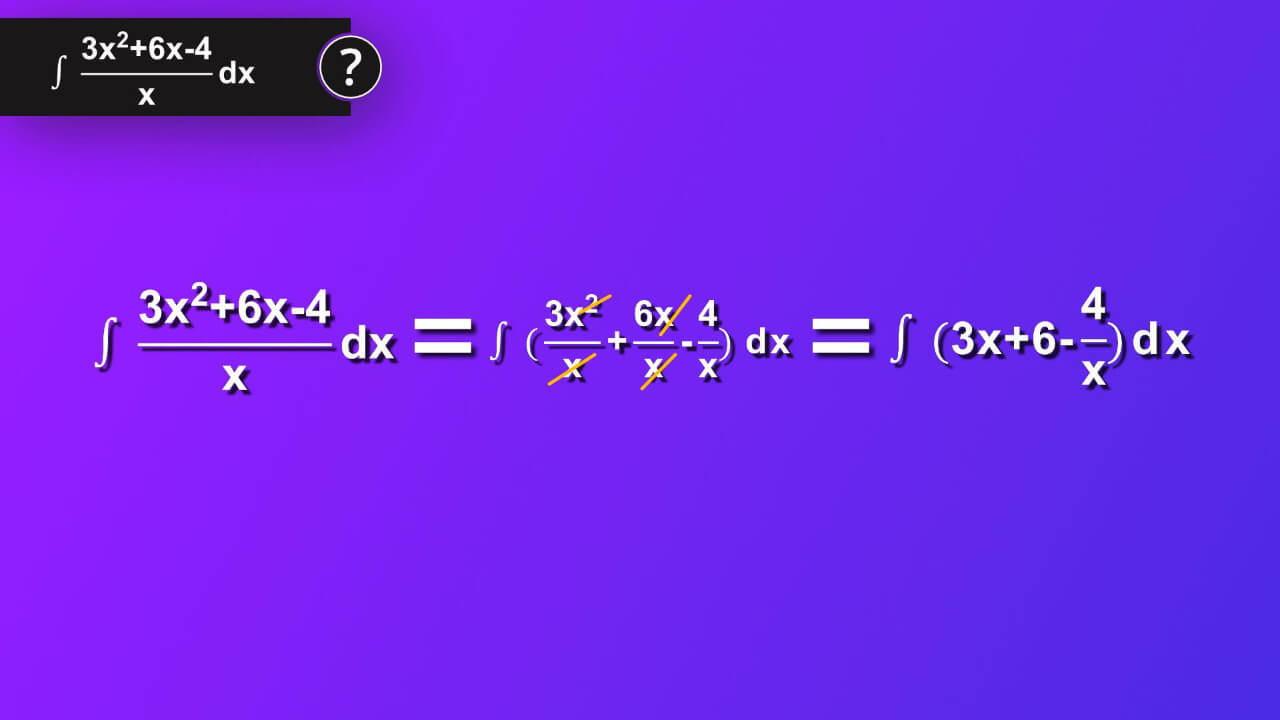### Step 4

Now, we want to take advantage of the formula which says in short that integral of sum of functions equals sum of separate integrals of each of those function.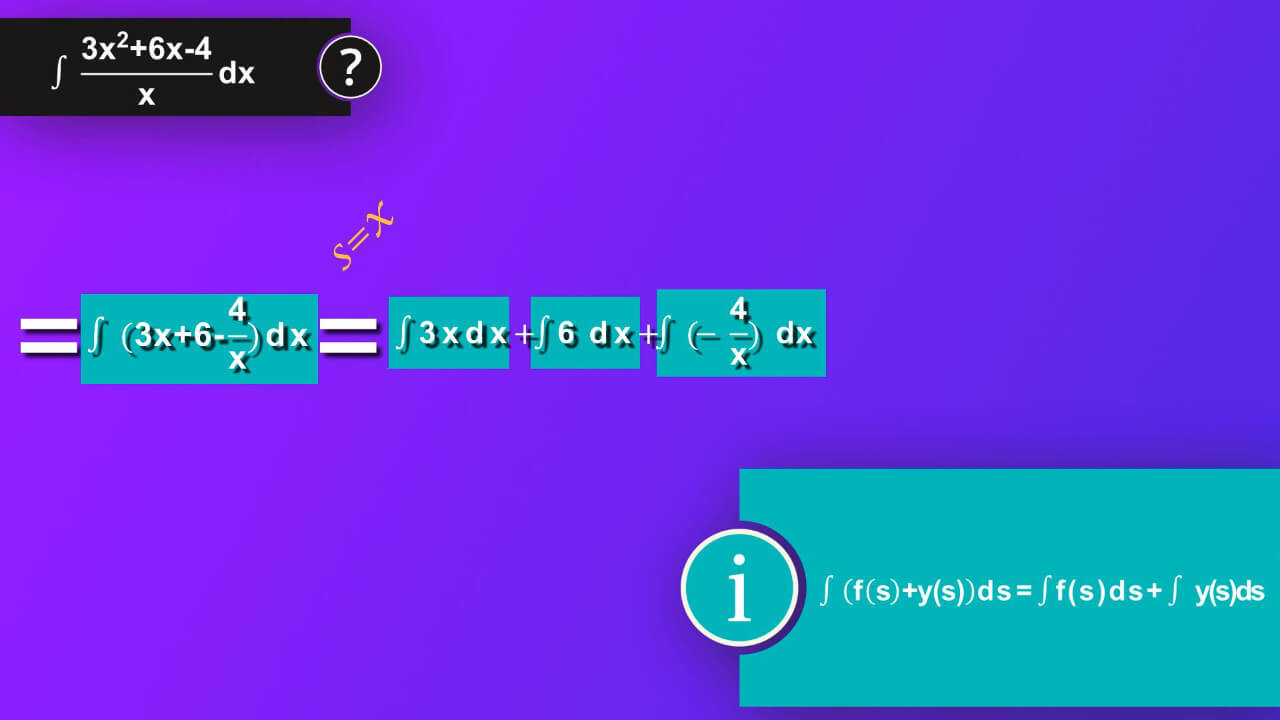### Step 5

Then, we can rewrite our equation as integral of 3 x by dx plus integral of 6 by dx plus integral of -4 divided by x by dx. Then, we can apply formula which says integral of constant a multiplied by variable s by ds equals constant a multiplied by integral of variable s by ds. In our case, we will apply it to each integral. First s is x and a is 3 and we’ve got 3 multiplied by integral of x by dx. Next, s is x rise to 0 or just 1 and a is 6 and we’ve got 6 multiplied by integral of dx. Finally, s is 1 divided by x and a is -4 and we’ve got -4 multiplied by integral of 1 divided by x by dx.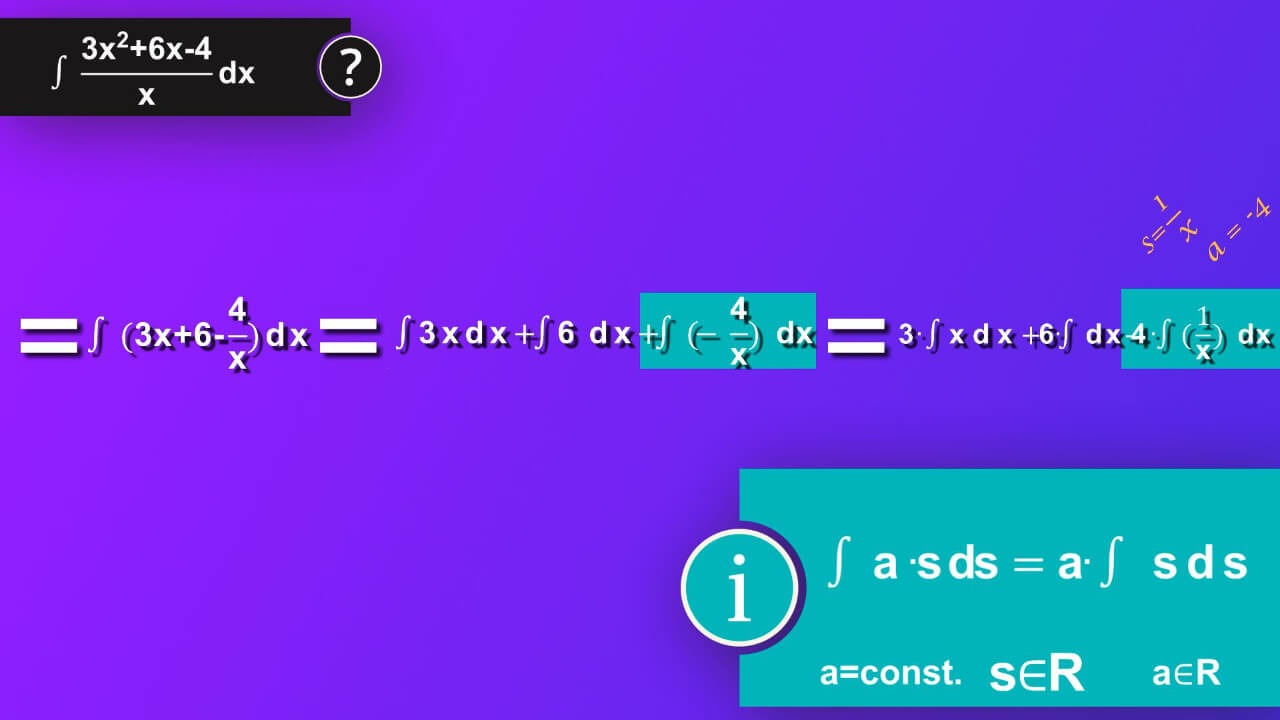### Step 6

Now it is time to solve each of our integral. To do so, we will use different formula for each integral. For the first one, we take advantage of formula which says integral of s rise to n by ds equals s rise to n+1 divided by n+1. We also must add constant C to make sure that we have all our solution taken into account as our solution make whole class of function not a single one. In case of first integral in our equation s is x and n is 1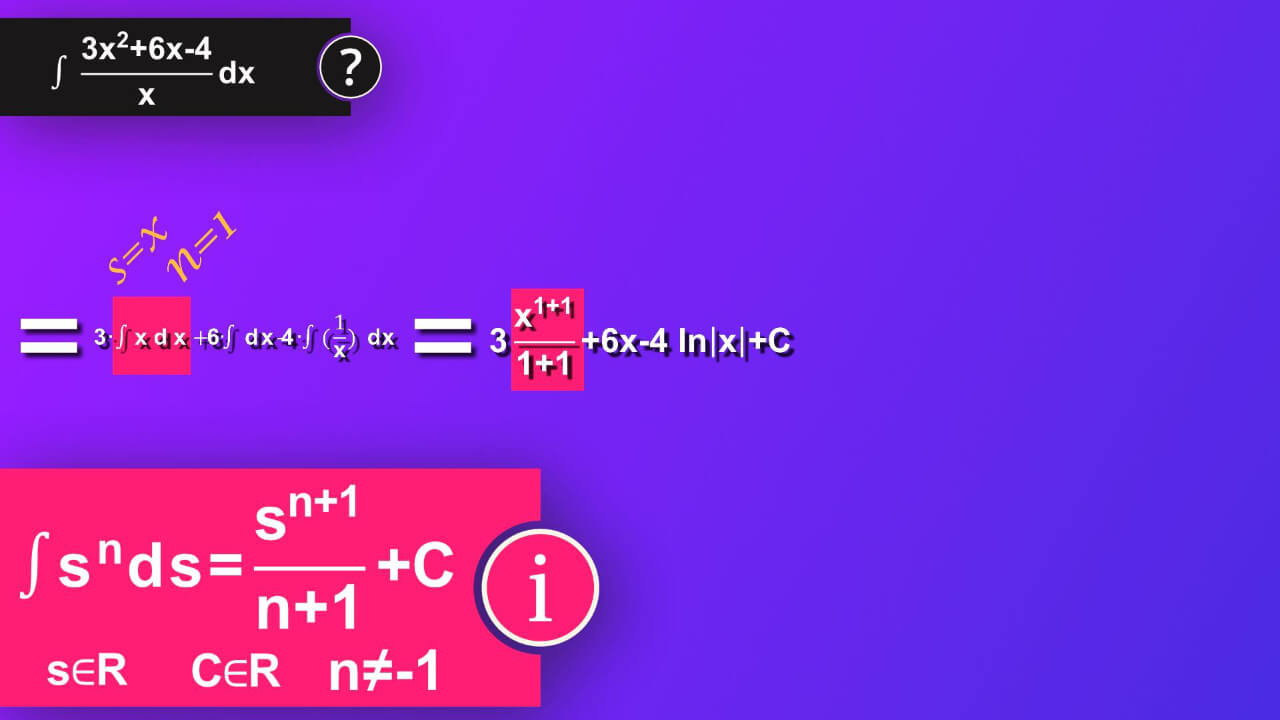### Step 7

and we’ve got 3 multiplied by x rise to 1+1 divided by 1+1.Second integral we will solve knowing that integral of ds equals s plus constant C. In or case s is x and we’ve got 6 multiplied by x in result. To the last integral we can apply formula which says integral of 1 divided by s by ds equals natural logarithm of absolute value of s plus constant C. In our case s is x and we’ve got minus 4 multiplied by natural logarithm of absolute value of x. And now we add constant C to our whole equation.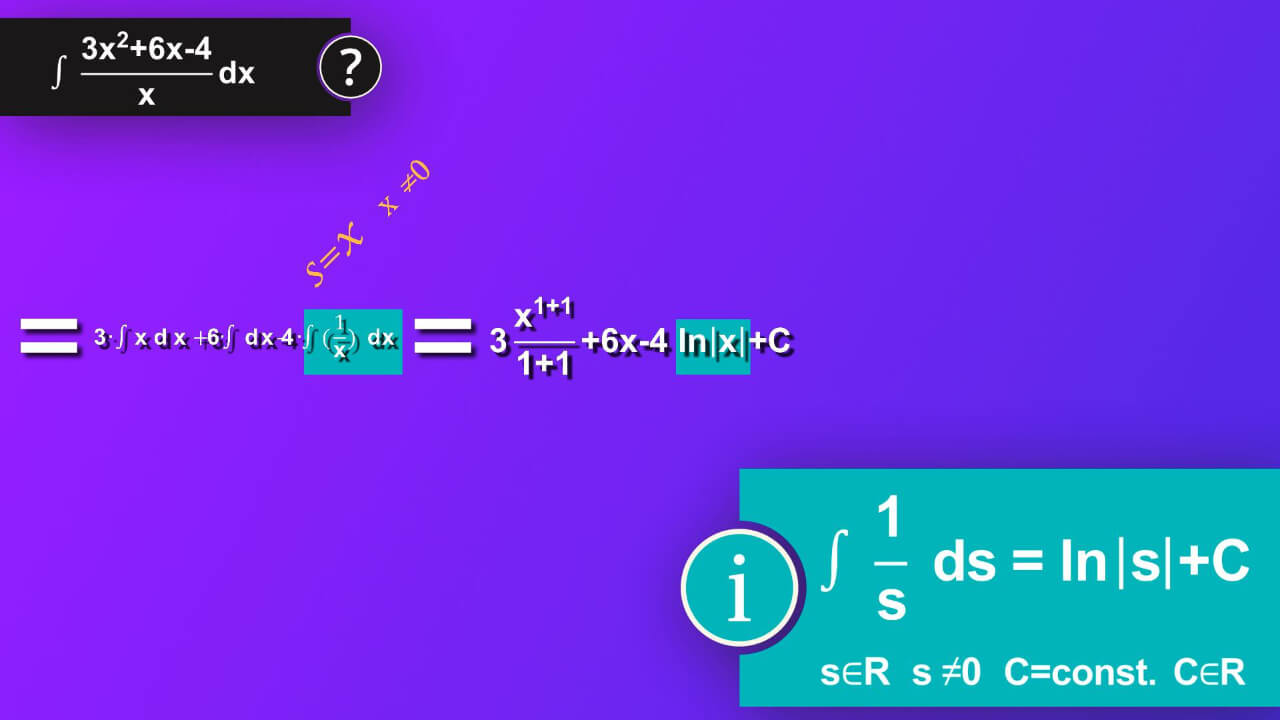### Step 8

In result, after smoothing, the solution is three-seconds multiplied by x square plus 6x minus 4 natural logarithms of absolute value of x plus constant C.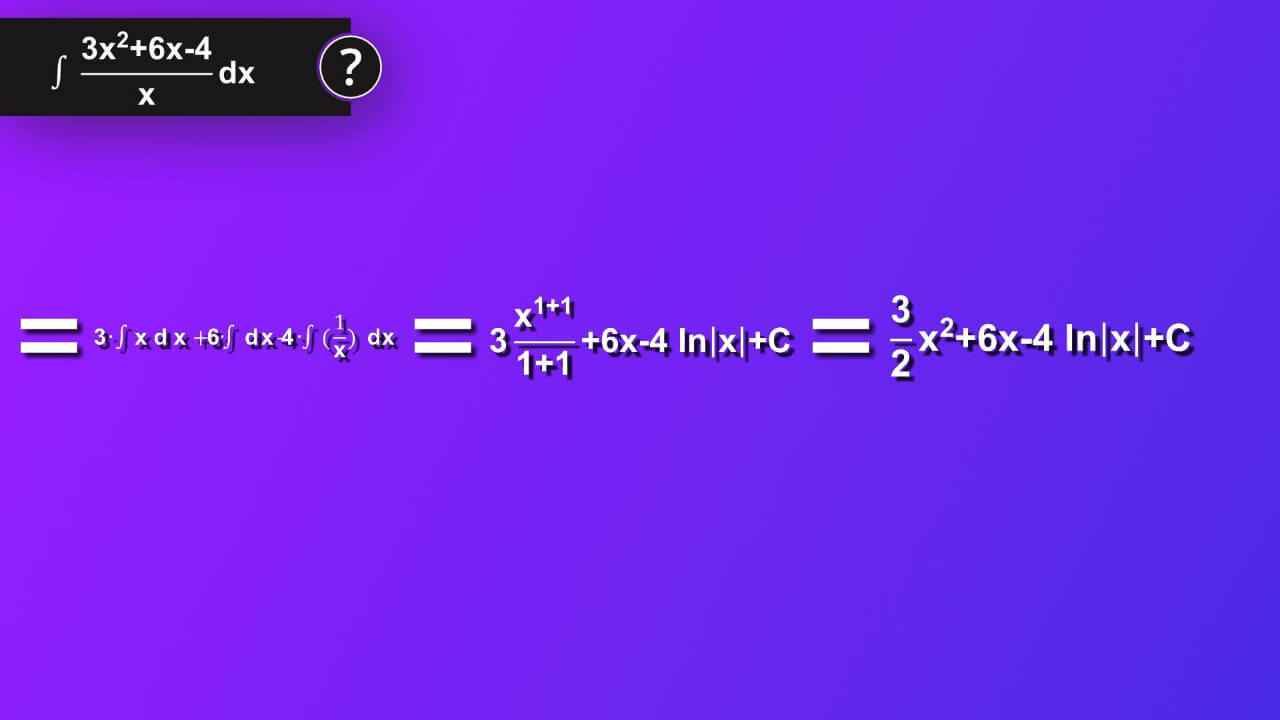## What is indefinite integral of 3 x square plus 6 x minus 4 divided by x by dx?

We finally did it: ∫ ((3x^2 +6x-4)/x)dx=98x+C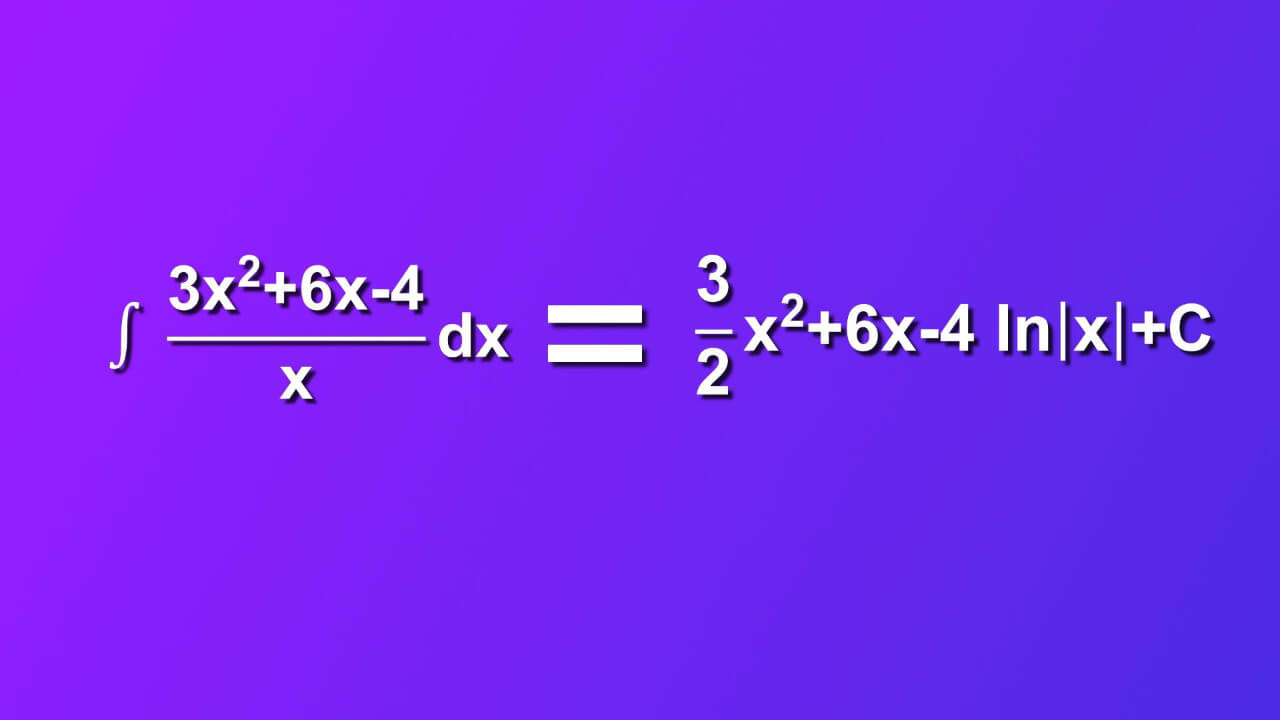## Dictionary

### Integration (antidifferentiation)

Computation (process of finding) of an integral, opposite process to differentiation.

### Integrand

Function placed between sign of integral and differential of variable of integration e.g. $${{ \int f(x)dx}}$$,
where:
$${{ \int }}$$- integration operator,
f(x) – integrand,
dx- differential of variable of integration x

### Integrable function

Function that integral over its domain is finite.

### Indefinite integral

Represents a class of primitive functions whose derivative is the integrand e.g. $${{\int f(x)dx=F(x)+C \Leftrightarrow F’(x)=f(x)}}$$
$${{C=const. }}$$,
$${{f, F,C \in R }}$$
R-real numbers
$${{\int f(x)dx}}$$ - indefinite integral of function f(x) by dx,
$${{F(x)+C}}$$ – a class of primitive functions that $${{F’(x)=f(x) }}$$,
F(x) - primitive function, usually written in capital letters,
R-real numbers.

### Function

Function specified on a set X and having values in set Y is an assignment each element of set X specifically one element in set Y.
$${{f: X \rightarrow Y}}$$
f-function name,
X-set of elements of function f, domain of a function f
Y-set of function values of function f, codomain of a function f
$${{x\in X, y \in Y}}$$
$${{y=f(x) f: x\rightarrow y}}$$
$${{y=y(x), }}$$
y(x)-vales of the function named y,
x-independent variable,
y-dependent variable.